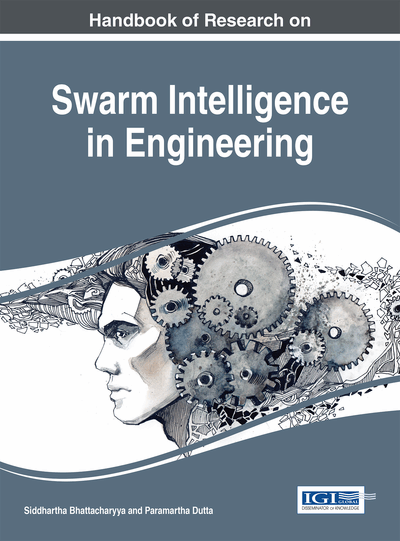# Minimax Probability Machine: A New Tool for Modeling Seismic Liquefaction Data

Pijush Samui (National Institute of Technology Patna, India), Yıldırım Huseyin Dalkiliç (Erzincan University, Turkey), Hariharan Rajadurai (VIT University, India) and J. Jagan (VIT University, India)
DOI: 10.4018/978-1-4666-8291-7.ch006
Available
\$37.50
No Current Special Offers

## Abstract

Liquefaction in soil is one of the other major problems in geotechnical earthquake engineering. This chapter adopts Minimax Probability Machine (MPM) for prediction of seismic liquefaction potential of soil based on Shear Wave Velocity (Vs) data. MPM has been used as a classification technique. Two models (MODEL I and MODEL II) have been adopted. In MODEL I, input variables are Cyclic Stress Ratio (CSR), and Vs MODEL II uses Peck Ground Acceleration (PGA) and Vs as input variables. The developed MPM has been compared with the Artificial Neural Network (ANN) and Support Vector Machine (SVM) models. The developed MPM is a robust tool for determination of liquefaction susceptibility of soil.
Chapter Preview
Top

## Introduction

Liquefaction of soil during earthquake is a major concern for the stability of civil engineering structure. Liquefaction is a phenomenon whereby a granular material transforms from a solid state to a liquefied state as a consequence of increase in pore water pressure. The effective stress of the soil therefore reduces causing loss of bearing capacity. Liquefaction of saturated sandy soils during the past earthquakes has resulted in building settlement and/or severe tilting, sand blows, lateral spreading, ground cracks, landslides, dam and high embankment failures and many other hazards. So, the determination of liquefaction susceptibility of soil is an important task in civil engineering. Liquefaction of soil depends on the following parameters:

• Intensity of earthquake and its duration,

• Location of ground water table,

• Soil type,

• Soil relative density,

• Particle shape,

• Depositional environment of soil,

• Soil drainage conditions,

• Confining pressures,

• Aging and cementation of the soil deposits,

• Historical environment of the soil deposit,

Civil engineers use different methods for determination of liquefaction susceptibility of soil (Seed & Idriss, 1971; Dobry et al., 1981; Seed et al., 1983; Seed & Idriss, 1982; Seed et al., 1985; Robertson & Campanella, 1983; Skempton, 1986; Seed & de-Alba, 1986; Stokoe et al., 1988a; Ambraseys, 1988; Tokimatsu & Uchida, 1990; Stark & Olson, 1995; Arango, 1996; Andrus & Stokoe, 1997; Youd & Noble, 1997a; Olsen, 1997; Robertson & Wride, 1998; Andrus & Stokoe, 2000; Moss et al., 2006). Liquefaction potential is evaluated by comparing equivalent measure of earthquake loading and liquefaction resistance. Earthquake loading characterization is generally done by using cyclic shear stress. By normalizing the cyclic shear stress amplitude by initial effective overburden stress, a cyclic stress ratio (CSR) is defined. CSR represents the level of cyclic loading induced at different depths in a soil profile, which corresponds to a specific earthquake. The resistance is mostly characterized based on field observation and potential for liquefaction is classified by comparing CSR with the liquefaction resistance, cyclic resistance ratio (CRR). There are different methods available for determination of liquefaction potential based on standard penetration test (SPT) (Seed & Idriss, 1967, 1971; Seed et al., 1983; Seed et al., 1984; Youd et al., 2001). These methods proposed boundary lines that separate field conditions causing liquefaction from conditions not causing liquefaction in sandy soils. Using this method, the CSR induced by the earthquake at any point in the ground is estimated as (Seed & Idriss, 1971).

(1) where τav = average equivalent uniform cyclic shear stress caused by the earthquake and is assumed to be 0.65 of the maximum induced stress; amax = peak horizontal ground surface acceleration; g = acceleration of gravity; σ'v= initial vertical effective stress at the depth in question; σv= total overburden stress at the same depth and rd= shear stress reduction coefficient to adjust for the flexibility of the soil profile and it has been estimated from the chart by Seed and Idriss (1971). The value of CSR is corrected to an earthquake magnitude of 7.5, using the magnitude correction (Cm) proposed by Seed et al. (1985). Seed et al. (1985) proposed a standard blow count N60. N60 has determined from the following relation:(2) where ER= percent of the theoretical free-fall energy; and N= SPT, N value corresponding to the ER. The value of N60 is corrected to an effective stress of 100 kPa. (N1)60 is obtained by using the following relation:(3) where CN is the effective stress correction factor and is calculated from the following relation:(4) where, Pa = 1atm of pressure in the same units used for σ′v

## Key Terms in this Chapter

Liquefaction: Liquefaction is the phenomenon in which the strength and the stiffness of the soil get reduced.

Minimax Probability Machine: It is defined as the process of building the classifiers by minimizing the maximum probability of misclassification.

Artificial Neural Network: Artificial Neural Networks are the modeling technique which was inspired from the central nervous system, capable of machine learning and pattern recognition.

Support Vector Machine: Support Vector Machines are supervised learning machine techniques that analyze data either for the prediction or classification purposes.

Classification: It is defined as the action of categorizing something.

Shear Wave Velocity: The velocity ( ? ) of a shear wave is equal to the square root of the ratio of shear modulus ( G ), to density ( ? ) of the medium, .

Prediction: Prediction is defined as the action of forecasting something earlier.

Earthquake: An earthquake is the sudden shaking of earth’s crust which leads to the great destruction in life and property.

## Complete Chapter List

Search this Book:
Reset